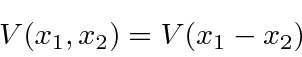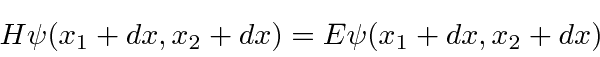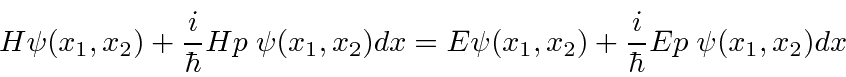## Quantum Mechanics for Two Particles

We can know the state of two particles at the same time. The positions and momenta of particle 2 commute with the positions and momenta of particle 1.The kinetic energy terms in the Hamiltonian are independent. There may be an interaction between the two particles in the potential. The Hamiltonian for two particles can be easily written.Often, the potential will only depend on the difference in the positions of the two particles.This means that the overall Hamiltonian has a translational symmetry. Lets examine an infinitesimal translation in. The original Schrödinger equationtransforms towhich can be Taylor expandedWe can write the derivatives in terms of the total momentum operator.Subtract of the initial Schrödinger equation and commutethrough.We have proven thatif the Hamiltonian has translational symmetry. The momentum is a constant of the motion. Momentum is conserved. We can have simultaneous eigenfunctions of the total momentum and of energy.

Jim Branson 2013-04-22